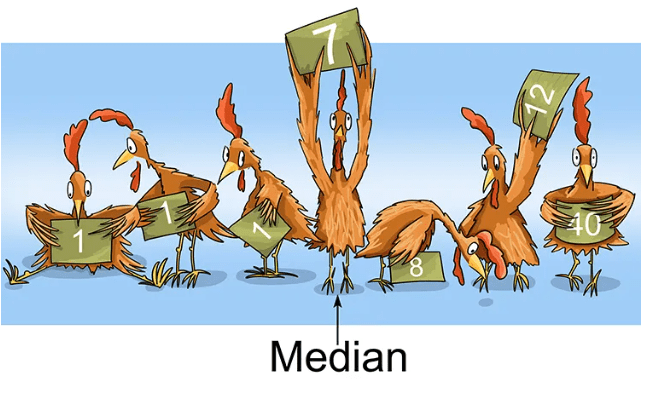# Median: Definition, Features, Examples, Pros & Cons

70 / 100

### Meaning of the Median

Median is the middle number of a set of numbers arranged in ascending or descending order of magnitude. If it is even, add the two middle numbers and divide the sum by two.

The median is a central position statistic that splits the distribution in two, that is, it leaves the same number of values on one side as on the other.To calculate the median it is important that the data are ordered from highest to lowest, or vice versa from lowest to highest. That is, they have an order.

For example, to find the median of 10, 17, 5, 18, 2, 17, 19 and 13, you need to arrange the scores in ascending order of magnitude as follows: 2, 5, 10, 13, 17, 17, 18, 19. The median of the scores from this arrangement is therefore:

13 + 17 / 2 = 30 / 2 = 15.

But if it is odd, then the formula for locating the median is to pick the most central figure when arranged in ascending order of magnitude.

The median, together with the mean and the variance, is a very illustrative statistic of a distribution. Unlike the mean, which can be shifted to one side or the other, depending on the distribution, the median is always located in the center of the distribution.

Incidentally, the shape of the distribution is known as kurtosis. With kurtosis we can see where the distribution is shifting.

### Formula for the Median

Once the median is defined, we are going to calculate it. For this, we will need a formula.

The formula will not give us the value of the median, what it will give us is the position in which it is within the data set. We must take into account, in this sense, if the total number of data or observations that we have (n) is even or odd. So the median formula is:

When the number of observations is even:

Median = (n+1) / 2 → Mean of the observations

When the number of observations is odd:

Median = (n+1) / 2 → Observation value

In other words, if we have 50 data ordered preferably from smallest to largest, the median would be in observation number 25.5. This is the result of applying the formula for an even data set (50 is an even number) and dividing by 2.

The result is 25.5 since we divided by 50+1. The median will be the mean between observation 25 and 26.

### Advantages of the use of Median

• It is simple to calculate and understand.
• Extreme items do not affect its value.
• It can be used for measuring qualities and factors to which a more rigorous mathematical measurement cannot be applied.
• It can be calculated from incomplete data.
• It can be determined from frequency diagrams.

### Disadvantages of the use of Median

• If the items are few, it is not likely to be a fair representative.
• The arrangement of data is often too tedious.
• It cannot be used to determine the total value of all the items.
• It is usually not representative of all the values in distribution.

### Examples of the Median

Suppose we have the following data:

2,4,12,6,8,14,16,10,18.

First of all we order them from smallest to largest with which we would have the following:

2,4,6,8,10,12,14,16,18.

Well, the value of the median, as indicated by the formula, is the one that leaves the same number of values both on one side and on the other. How many observations do we have?

9 observations.

We calculate the position with the corresponding median formula.

Median = 9+1 / 2 = 5

What does this 5 mean? It tells us that the value of the median is found in the observation whose position is the fifth.

Therefore the median of this would be the number 10, since it is in the fifth position. In addition, we can check how to the left of 5 there are 4 values (2, 4, 6 and 8) and to the right of 10 there are another 4 values (12, 14, 16 and 18).